# Pandas Select rows by condition and String Operations

There are instances where we have to select the rows from a Pandas dataframe by multiple conditions. Especially, when we are dealing with the text data then we may have requirements to select the rows matching a substring in all columns or select the rows based on the condition derived by concatenating two column values and many other scenarios where you have to slice,split,search substring with the text data in a Pandas Dataframe. Here we are going to discuss following unique scenarios for dealing with the text data:

• Select all rows containing a sub string
• Select rows by list of values
• Select rows by multiple conditions
• Select rows by index condition
• Select rows by list of index
• Extract substring from a column values
• Split the column values in a new column
• Slice the column values
• Search for a String in Dataframe and replace with other String
• Concat two columns of a Dataframe

Search for String in Pandas Dataframe

Let’s create a Dataframe with following columns: name, Age, Grade, Zodiac, City, Pahun

``````import pandas as pd
df = pd.DataFrame({'name':['Allan2','Mike39','Brenda4','Holy5'], 'Age': [30,20,25,18],'Zodiac':['Aries','Leo','Virgo','Libra'],'Grade':['A','AB','B','AA'],'City':['Aura','Somerville','Hendersonville','Gannon'], 'pahun':['a_b_c','c_d_e','f_g','h_i_j']})
``````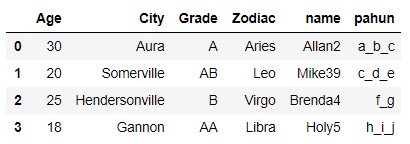We will select the rows in Dataframe which contains the substring “ville” in it’s city name using str.contains() function

``````df[df.City.str.contains('ville',case=False)]
``````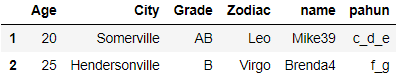Select rows by list of values

We will now select all the rows which have following list of values ville and Aura in their city Column

``````search_values = ['ville','Aura']
``````
``````df[df.name.str.contains('|'.join(search_values ))]
``````

After executing the above line of code it gives the following rows containing ville and Aura string in their City name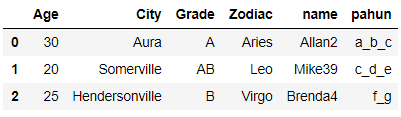Filter rows by Multiple Conditions

We will select all rows which has name as Allan and Age > 20

``````df[(df.Age.isin([30,25])) & (df.name.str.contains('Allan'))]
``````

Select row by index

We will see how we can select the rows by list of indexes. Let’s change the index to Age column first

``````df.set_index(df.Age,inplace=True)
``````

Now we will select all the rows which has Age in the following list: 20,30 and 25 and then reset the index

``````df.loc[[20,30,25]].reset_index(drop=True)
``````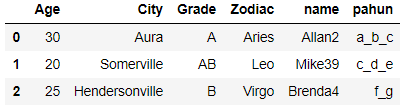Extract number from String

The name column in this dataframe contains numbers at the last and now we will see how to extract those numbers from the string using extract function. We will use regular expression to locate digit within these name values

``````df.name.str.extract(r'([\d]+)',expand=False)
``````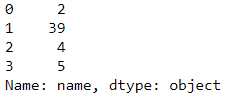We can see all the number at the last of name column is extracted using a simple regular expression

Remove Number from String

In the above section we have seen how to extract a pattern from the string and now we will see how to strip those numbers in the name

``````df['name']=df.name.str.replace('\d+', '')
``````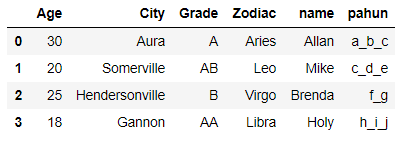The name column doesn’t have any numbers now

String Split and create new columns

The pahun column contains the characters separated by underscores(_). We will split these characters into multiple columns

``````df[['pahun_1','pahun_2','pahun_3']]=df['pahun'].str.split('_', expand=True,n=2)
``````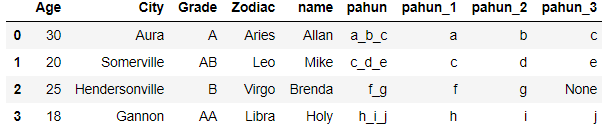The Pahun column is split into three different column i.e. pahun_1,pahun_2,pahun_3 and all the characters are split by underscore in their respective columns

String Slice

Lets create a new column (name_trunc) where we want only the first three character of all the names. so for Allan it would be All and for Mike it would be Mik and so on. The string indexing is quite common task and used for lot of String operations

``````df['name_trunc']=df.name.str.slice(start=0, stop=3, step=1)
``````

OR

``````df['name_trunc']=df.name.str[:3]
``````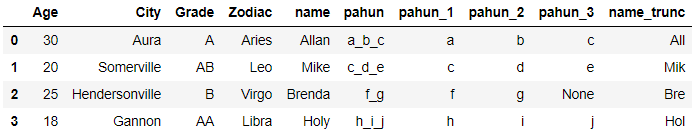The last column contains the truncated names

Search String with Patterns

We want to now look for all the Grades which contains A

``````import re
``````

This will give all the values which have Grade A so the result will be a series with all the matching patterns in a list. for example: for the first row return value is [A]

Pandas Concat Columns

We have seen situations where we have to merge two or more columns and perform some operations on that column. so in this section we will see how to merge two column values with a separator

We will create a new column (Name_Zodiac) which will contain the concatenated value of Name and Zodiac Column with a underscore(_) as separator

``````df['name_zodiac'] = df.name.str.cat(df.Zodiac,sep='_')
``````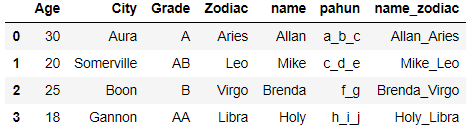The last column contains the concatenated value of name and column

Conclusion

So you have seen Pandas provides a set of vectorized string functions which make it easy and flexible to work with the textual data and is an essential part of any data munging task. These functions takes care of the NaN values also and will not throw error if any of the values are empty or null.There are many other useful functions which I have not included here but you can check their official documentation for it.

Tags:

Categories:

Updated: